# Precalculus : Angular and Linear Velocity

## Example Questions

2 Next →

### Example Question #1 : Solve Linear Velocity Problems

A clock has a second hand measuring 12cm. What is the linear speed of the tip of the second hand?

Possible Answers: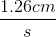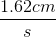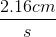None of these/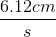Correct answer:Explanation:

Linear speed is equal to the arc length traversed divided by the time. We use s to denote arc length.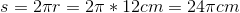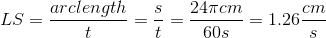2 Next →

### All Precalculus Resources